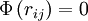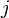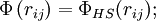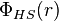2022: SklogWiki celebrates 15 years on-line

# Widom-Rowlinson model

(diff) ← Older revision | Latest revision (diff) | Newer revision → (diff)$\Phi \left( r_{ij} \right) = 0$; if$i$ and$j$ are particles of the same species$\Phi \left( r_{ij} \right) = \Phi_{HS}(r_{ij});$ if$i$ and$j$ belong to different species,
where$\Phi_{HS}(r)$ is the hard sphere intermolecular pair potential.